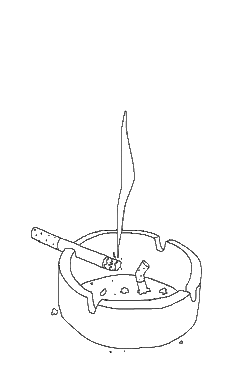# April 2020xxxxxxxxxxx
Page 2xx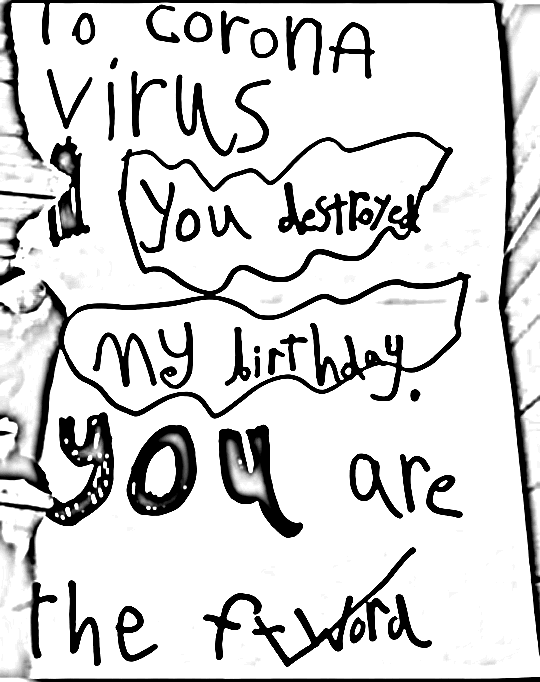xxxPage 3x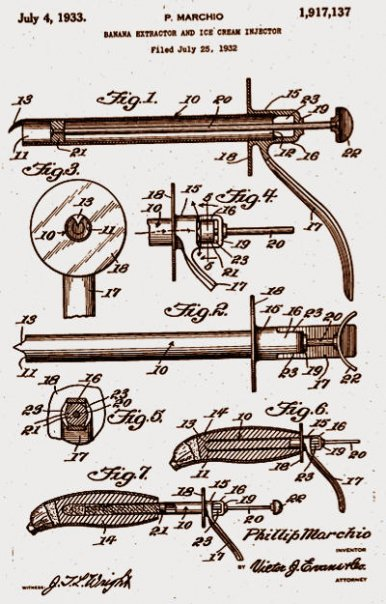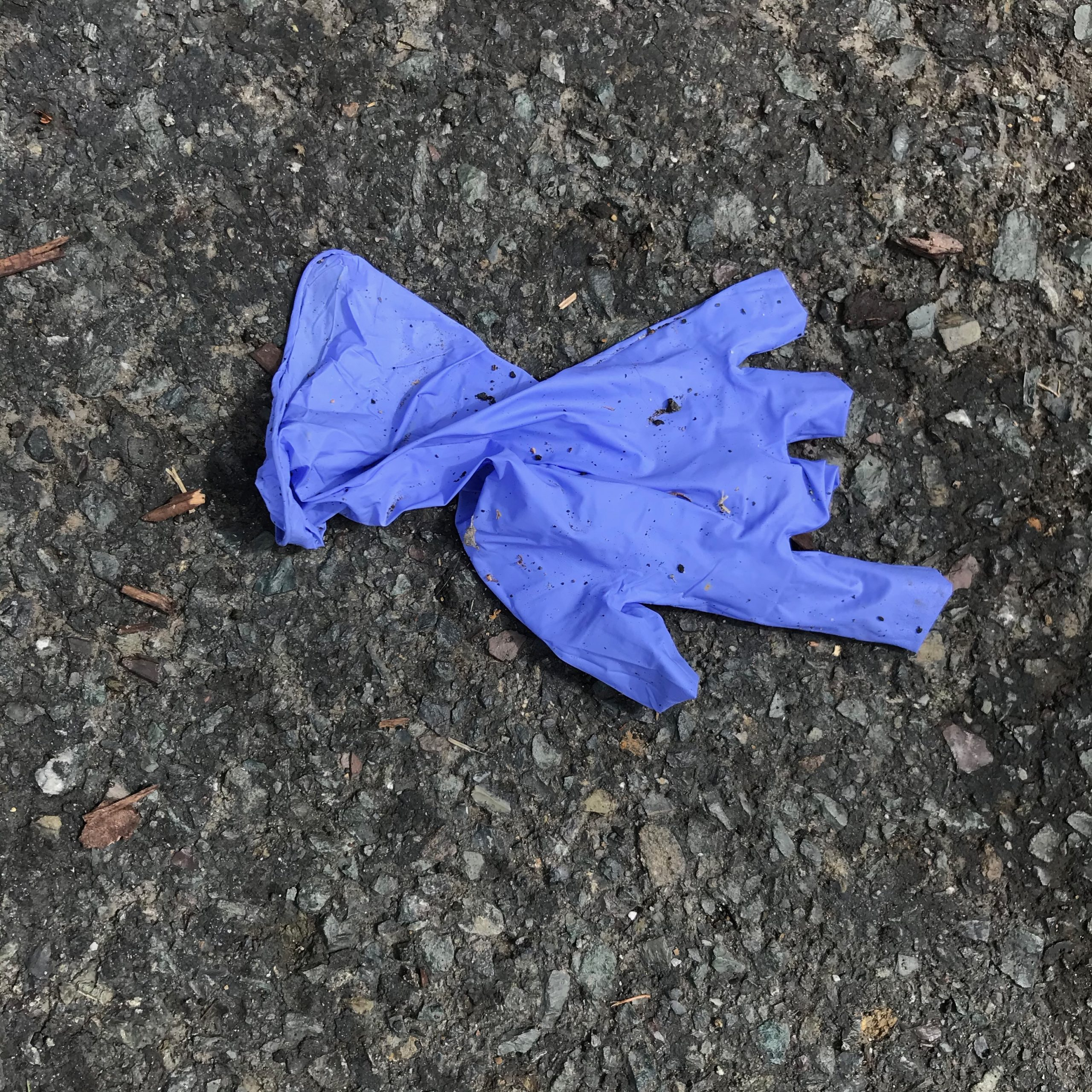x
Page 4xxxxx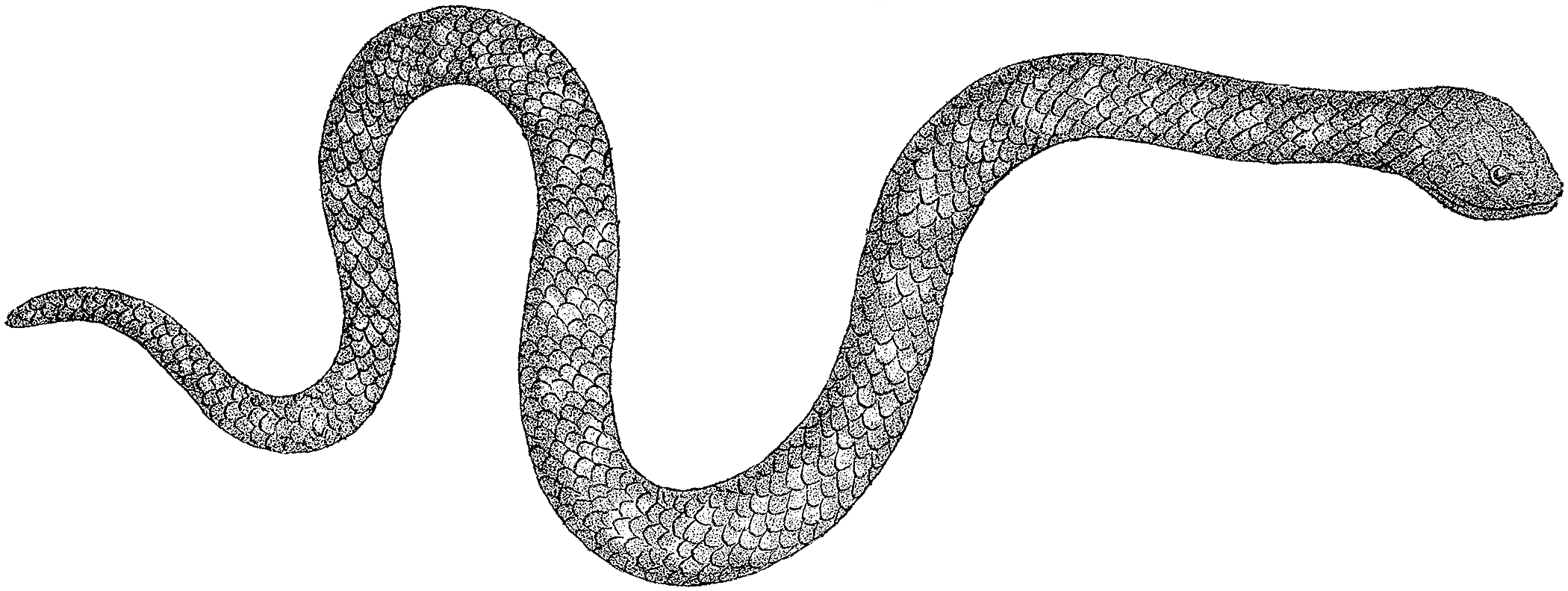xxx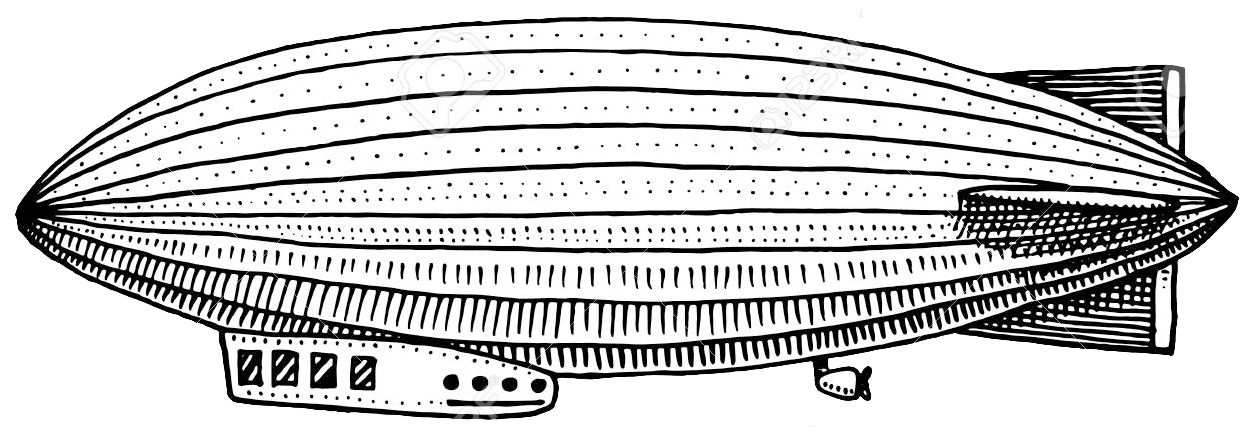xPage 5xx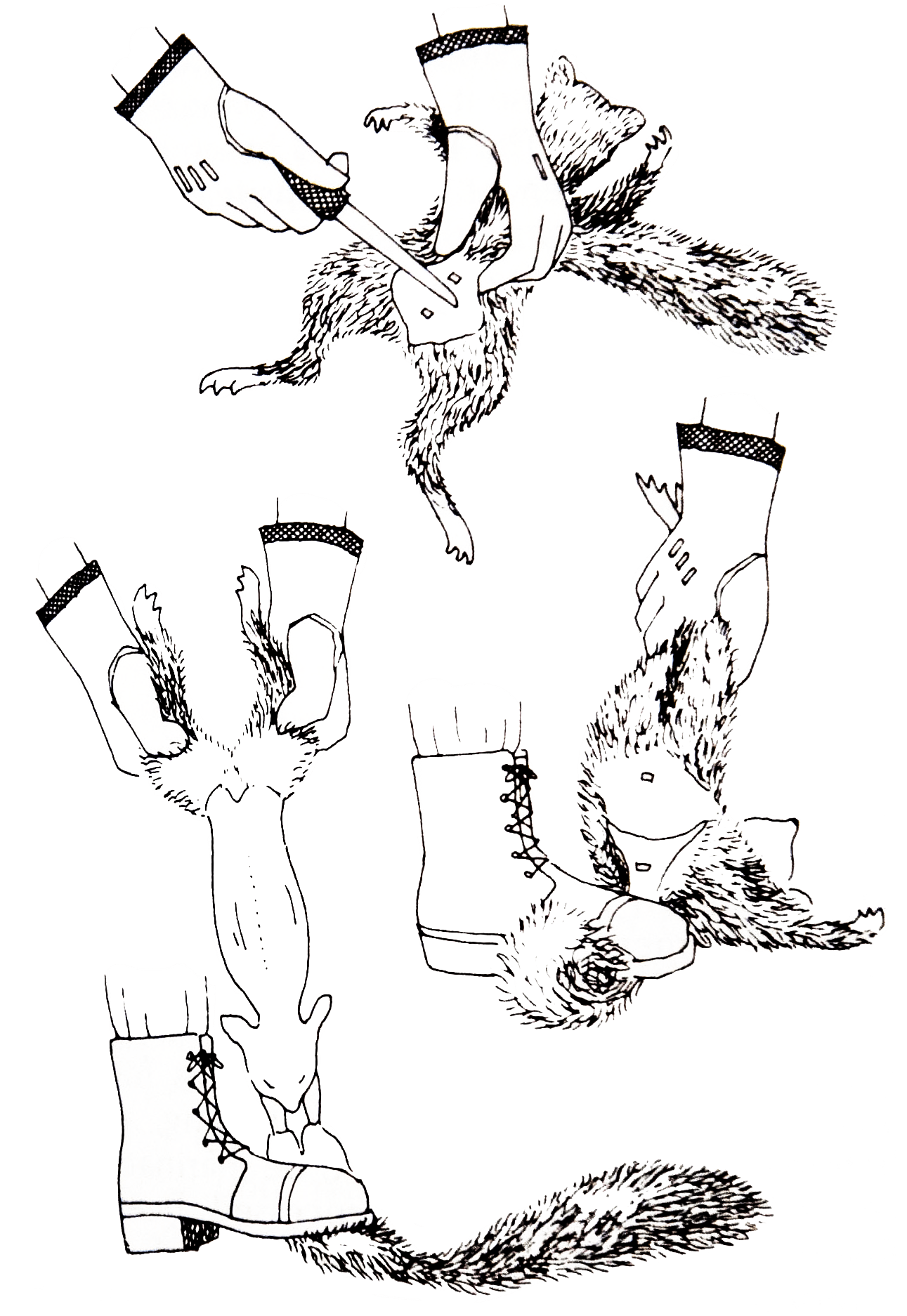xxx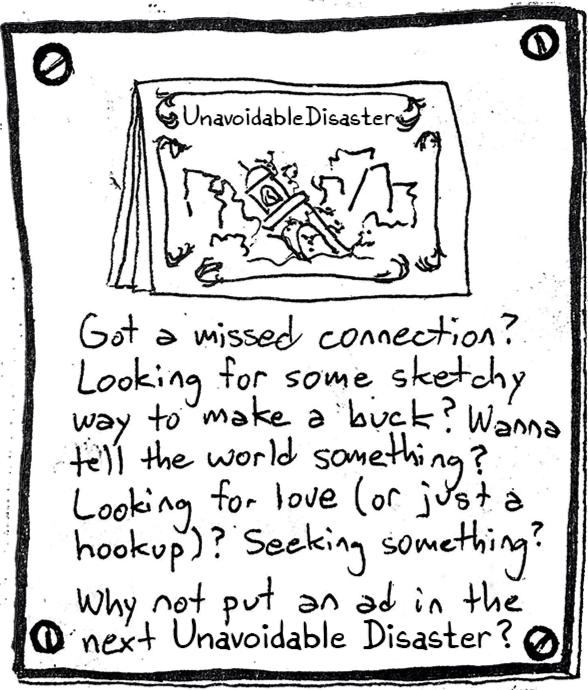xPage 6